# phot – photometry/flux measurement classes and tools¶

The phot module contains classes and functions for photometry or other image-based flux measurements.

## Classes and Inheritance Structure¶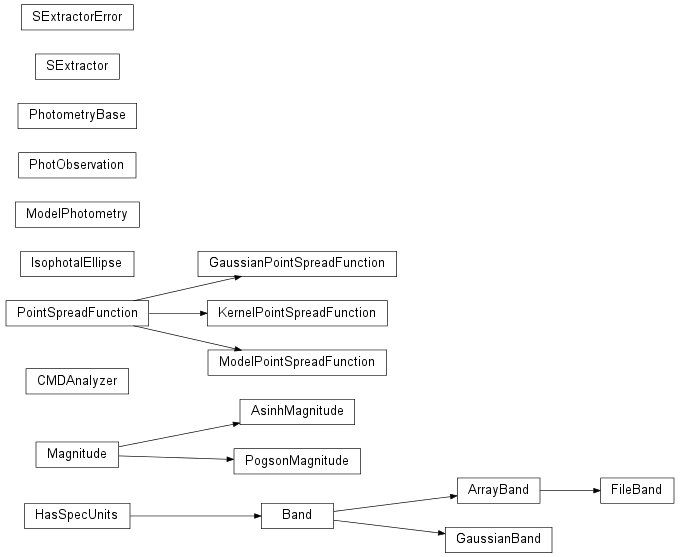## Module API¶

class astropysics.phot.ArrayBand(x, S, copyonaccess=True, normalized=True, unit='angstroms', name=None)

Bases: astropysics.phot.Band

generate a Band from a supplied quantum (e.g. photonic) response function

if the response function is ever negative, the band will be offset so that the lowest point is 0 . If the band x-axis is not sorted, it will be sorted from lowest to highest.

normalized
class astropysics.phot.AsinhMagnitude(b=1e-10)
a = -1.0857362047581294
b
fluxToMag(flux)
fluxerrToMagerr(err, flux)
magToFlux(mag)
magerrToFluxerr(err, mag)
class astropysics.phot.Band

This class is the base of all photometric band objects. Bands are expected to be immutable once created except for changes of units.

The response functions should be photon-counting response functions (see e.g. Bessell et al 2000).

Subclasses must implement the following methods:

• __init__()

initialize the object

• _getCen()

return the center of band

• _getFWHM()

return the FWHM of the band

• _getx()

return an array with the x-axis

• _getS()

return the photon sensitivity (shape should match x)

• _applyUnits()

units support (see astropysics.spec.HasSpecUnits)

the following can be optionally overriden:

• alignBand()

return the sensitivity of the Band interpolated to the provided x-array

• alignToBand():

return the provided sensitivity interpolated onto the x-array of the Band

FWHM
S
alignBand(x, interpolation='linear')

interpolates the Band sensitivity onto the provided x-axis

x can either be an array with the wanted x-axis or a Spectrum if it is a spectrum, the units for the band will be converted to the Spectrum units before alignment

interpolation can be ‘linear’ or ‘spline’

alignToBand(*args, **kwargs)

Interpolates the provided function onto the Band x-axis. Input arguments can be of the following forms:

• alignToBand(arr)

assume that the provided sequence fills the band and interpolate it onto the Band coordinates

• alignToBand(specobj)

if specobj is of type astropyics.spec.Spectrum, the spectrum’s flux will be returned aligned to the band, in the units of the Band

• alignToBand(x,y)

the function with coordinates (x,y) will be aligned to the Band x-axis

keywords
• interpolation

see alignBand() for interpolation types/options

cen
computeFlux(spec, interpolation='linear', aligntoband=None, overlapcheck=True)

compute the flux in this band for the supplied Spectrum

the Spectrum is aligned to the band if aligntoband is True, or vice versa, otherwise, using the specified interpolation (see Band.alignBand for interpolation options). If aligntoband is None, the higher of the two resolutions will be left unchanged

if overlapcheck is True, a ValueError will be raised if most of the Spectrum does not lie within the band

the spectrum units will be converted to match those of the Band

the spectrum can be an array, but then aligntoband and interpolation are ignored and must match the band’s x-axis

computeMag(*args, **kwargs)

Compute the magnitude of the supplied Spectrum in this band using the band’s zptflux attribute

args and kwargs go into Band.computeFlux

computeZptFromSpectrum(*args, **kwargs)

use the supplied Spectrum as a zero-point Spectrum to define the zero point of this band as well as the flux for that zero-point

args and kwargs are the same as those for computeMag

isOverlapped(xorspec)

determes if this Band overlaps on a significant part of the provided spectrum (that is, if center+/-fwhm overlaps)

name
plot(spec=None, bandscaling=1, bandoffset=0, labelband=True, clf=True, **kwargs)

this plots the requested band and passes kwargs into matplotlib.pyplot.plot OR if spec is provided, the spectrum will be plotted along with the band and the convolution

keywords

• clf

clear the figure before plotting

• leg

show legend where appropriate

source
x
zptflux = 1
class astropysics.phot.CMDAnalyzer(fiducial, fbands, fidname='fiducial')

Bases: object

This class is intended to take multi-band photometry and compare it to fiducial models to find priorities/matches for a set of data from the same bands

fiducial is a B x N array where B is the number of bands/colors and N is the number of fiducial points.

fbands specifies the band (either as a string or a Band object) or color name (seperated by “-”) matching the fiducial

fidname is a string or a dictionary that maps fiducial names to indecies in the fidcuial array. If a dictionary, each index must be in one of the values (but not in more than one)

bandnames
center

The center of the data for prioritizing based on distance

clearOffsets()

clears the offsets for setting of properties without recalculation

data

see getData and setData

datagroups

Group numbers for the objects - will be cast to ints. Typical meanings: 0 -> don’t use in spectrum -1 -> alignment or guide star

datanames

Names for the objects - default is in numerical order starting at 1

distance

The distance to use in calculating the offset between the fiducial values and the data

distmod

The distance modulusto use in calculating the offset between the fiducial values and the data

fidnames
getBand(i)
getData(i=None)
getFiducialData(i, fidname=None)

Retreives fiducial data values in a particular band.

i is either an index into the bands, or a band name.

fidname specifies which fiducial sequence to use (or if None, the full array for the requested band will be returned)

getOffsets()

computes and returns the CMD offsets

locs

The physical location of the data for prioritizing based on distance

locweight

Weights to apply to the location while calculating the offset.

offsetbands

this selects the bands or colors to use in determining offsets as a list of band names/indecies (or for colors, ‘b1-b2’ or a 2-tuple) if None, all the bands will be used directly.

offsetweights

Weights to apply to bands while calculating the offset. Note that this will be invalidated whenever the offsetbands change

if None, no weighting will be done. otherwise, offsetweights must be a sequence of length equal to offsetbands. positibe values will be interpreted as raw scalings (i.e. offset=w*(dat-fid) ) and negative values will be compared to the total range (i.e. offset=-w*(dat-fid)/(max-min) )

plot(bx, by, clf=True, skwargs={}, lkwargs={})

this plots the two bands specified by bx and by

clf determines if the figure should be cleared before plotting

skwargs are passed into pylab.scatter and lkwargs are passed into pylab.plot

setData(val)

This loads the data to be compared to the fiducial sequence - the input can be an array (B X N) or an

validbandinds
validbandnames
class astropysics.phot.FileBand(fn, type=None)

type can be ‘txt’, or ‘fits’, or inferred from extension

if txt, first column should be lambda, second should be response

class astropysics.phot.GaussianBand(center, width, A=1, sigs=6, n=100, unit='angstroms', name=None)

Bases: astropysics.phot.Band

center is the central wavelength of the band, while width is the sigma (if positive) or FWHM (if negative)

alignBand(x)
n
sigma
sigs
convolve(arr2d, background=0)
fit(arr2d)

fits the image using a moment analysis - returns (cenx,ceny),[sigx,sigy]

fwhm
class astropysics.phot.IsophotalEllipse(imdata, isolevel=None)

Bases: object

Generates best fit ellipses for an image

a

returns major axis length in image coordinates

axisratio

returns major/minor axis ratio

b

returns major axis length in image coordinates

cartesian(npoints)

returns the full ellipse as an (x,y) tuple

e

returns eccentricity

fixcenter
getDiff(fractional=False)

returns the difference between the fitted ellipse and the flux level

imdata
isolevel
keeppix

The pixels to keep for fitting, either as a fraction of the total (if less than 1) or as a fixed number of pixels (if greater than 1)

major

returns major axis length in image coordinates

minor

returns major axis length in image coordinates

nfitpoints
phi

returns rotation angle clockwise from x-axis

plot(clf=True)
polar(npoints)

returns the full ellipse as an (rho,theta) tuple

convolve(arr2d, background=0)
fit(arr2d)
kernel
astropysics.phot.ML_ratio_from_color(c, color='B-V')

uses Bell&DeJong 01 relations - note that this is intended for normal spiral stellar pops

color can either be a ‘B-V’,’B-R’,’V-I’,’V-J’,’V-H’,or ‘V-K’

returns tuple of mass-to-light ratios for each c as (mlrb,mlrv,mlrr,mlri,mlrJ,mlrH,mlrK)

astropysics.phot.ML_ratio_from_color_SDSS(c, color='g-r')

Uses Bell 2003 relations derived from SDSS to compute the mass-to-light ratio of a galaxy goven it’s color

Parameters: c (float or array-like) – The color of the galaxy (or galaxies) in magnitudes color (string) – The colors of input c - can be ‘u-g’, ‘u-r’, ‘u-i’, ‘u-z’, ‘g-r’, ‘g-i’, ‘g-z’, ‘r-i’, or ‘r-z’. tuple of mass-to-light ratios in each band (mlrg,mlrr,mlri,mlrz,mlrJ,mlrH,mlrK) for each color.
astropysics.phot.M_star_from_mags(B, V, R, I, color='B-V')

Uses Bell & DeJong 2001 relations to compute stellar mass - note that this is intended for normal spiral stellar pops.

Parameters: B (float or array-like) – B-band absolute magnitude. V (float or array-like) – V-band absolute magnitude. R (float or array-like) – R-band absolute magnitude. I (float or array-like) – I-band absolute magnitude. color (string) – The color to use for computing the stellar mass - either ‘B-V’, ‘B-R’, ‘V-I’, or ‘mean’. Stellar mass derived using the each possible color as well as the mean, in a tuple (meanmstar,(B-derived,V-derived,R-derived,I-derived)).
astropysics.phot.M_star_from_mags_SDSS(u, g, r, i, z, J=None, H=None, K=None, color='mean')

Uses Bell 2003 relations derived from SDSS - computes stellar mass given magnitudes for a galaxy.

class astropysics.phot.Magnitude

Bases: object

fluxToMag(flux)
fluxerrToMagerr(err, flux)
magToFlux(mag)
magerrToFluxerr(err, mag)
class astropysics.phot.ModelPhotometry(model, magzpt=0)

Bases: object

Represents a astropysics.models 2D model of the photometric flux from an image.

The normparam attribute defines which model parameter sets the overall scaling. If None, the scaling methods will not function.

fitPhot(im)

computes the radius in which half the flux is encircled. kwargs are passed into FunctionModel2DScalar.getFluxRadius

model
normparam = ('A', 'Ae')
simulate(pixels, scale=1, background=0, noise=None, psf=None, sampling=None)

Simulate how this model would appear on an image.

pixels is a 2D array that described the number of pixels to use, a scalar describing the number of pixels in both directions, or a sequence (npixx,npixy)

scale is either a scalar giving the pixel scale or a 2-sequence (xscale,yscale) as units/pixel

background is a scalar background level to assume or a 2D array of matching shape as the simulation

noise can be:

• None

no noise is applied

• ‘poisson’

assumes the flux units are counts and returns the poisson noise model

• a scalar

uniform gaussian noise with the scalar as sigma

• a 2d array

gaussian noise with the value at each array point as sigma - array dimension must match simulation

• a callable

will be called as noise(imagearr2d) and should return the value of the model+noise

psf is the point-spread function to be applied before the noise, either a scalar/2-tuple for a gaussian PSF (specifies the FWHM in actual units, not pixels), or a 2D measured PSF to convolve with the model. If None, no psf will be included.

sampling is passed into the model’s pixelize method (see FunctionModel2DScalar.pixelize())

totalflux
totalmag
convolve(arr2d, background=False)
fit(arr2d, **kwargs)

kwargs are passed into the model fitData method

model
class astropysics.phot.PhotObservation(bands, values, errs=None, asmags=True)

Bases: object

A photometric measurement (or array of measurements) in a fixed group of bands. These bands must be present in the band registry.

the first index of the values must have length equal to nbands

if asmags is True, values and errs will be interpreted as magnitudes, otherwise, flux

bandnames
bands
flux

photmetric measurements in flux units

fluxerr

photmetric errors in flux units

getBandInfo(unit='angstroms')

Extracts the center and FWHM of the bands in these observations

returns x,w

getFluxDensity(unit='angstroms')

compute an approximate flux density (e.g. erg cm^-2 s^-1 angstrom^-1) assuming flat over the FWHM

mag

photmetric measurements in magnitudes

magerr

photmetric errors in magnitudes

plot(includebands=True, fluxtype=None, unit='angstroms', clf=True, **kwargs)

Plots these photometric data points.

fluxtype can be:

• None

either magnitude or flux depending on the input type

• ‘mag’

magnitudes

• ‘flux’

flux in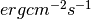• ‘fluxden’

flux spectral density e.g.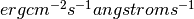kwargs go into matplotlib.pyplot.errorbar

toSpectrum(unit='angstroms')

converts this data set to a Spectrum

TODO: fill in gaps assuming a flat spectrum

class astropysics.phot.PhotometryBase

Bases: object

A base class for objects that perform photometric measurements from an image.

Subclasses should:

• override _compute to take an image and perform the computation steps necessary to generate the parameters
• pass any outputs through _fluxToMag to do flux>mag conversion

all outputs will be in magnitudes with the zeropoint set by the zeropoint attribute if usemags is True, otherwise they will be

band
computePhotometry(**kwargs)

Performs the primary computationally-intensive phase of the photometric measurement.

kwargs will be set as attributes on this object

image = None
loc = None
psf = None
totalflux
totalmag
zeropoint

If set to None, zeropoint comes from image

class astropysics.phot.PogsonMagnitude
a = -1.0857362047581294
fluxToMag(flux)
fluxerrToMagerr(err, flux)
magToFlux(mag)
magerrToFluxerr(err, mag)

Bases: object

Represents a Point Spread Function (PSF) for an image.

Coordinate system is always in pixel coordinates

convolve(arr2d, background=0)

Convolve this psf with the supplied 2D Array.

background sets the value of the background to assume around the edges.

fit(arr2d)

set the PSF parameters from the provided 2D image array

class astropysics.phot.SExtractor(sexfile=None, parfile=None)

Bases: object

This class is an adaptor to the Sextractor program (Bertin & Arnouts 1996, http://astromatic.iap.fr/software/sextractor/). Sextractor must be installed and on the path for this class to function.

options are set by changing values in the options dictionary

output parameters are chosen by setting True/False values in the params dictionary

static getOptInfo(aslist=False)

returns the dictionary of input options and the associated information

if aslist is True, returns an list of

getOptionList(incval=False)

returns a list of all options. If incval is True, returns a list of tuples of the form (optionname,optionvalue)

static getParamInfo(aslist=False)

returns the dictionary of parameters and the associated information

getParamList()

returns a list of all selected parameters

sextractImage(detimfn, analysisimfn=None, mode='waiterror')

writes configuration files and runs sextractor on the input image

mode can be:

• ‘waiterror’: waits for sextractor to finish, and raises an SExtractorError if it does not complete sucessfully. stdout and sterr are saved to self.lastout and self.lasterr (returns 0)
• ‘wait’: waits for sextractor to finish and returns the return code stdout and sterr are saved to self.lastout and self.lasterr
• ‘proc’: stars the processes but does not wait - returns the Popen instance of the processes
exception astropysics.phot.SExtractorError

Bases: exceptions.Exception

astropysics.phot.UBVRI_to_ugriz(U, B, V, R, I, ugrizprimed=False)

This function transforms UBVRI (Johnson-Cousins) photometry to the SDSS system, using the transformations of Jester et al. (2005). If only UBV is available, only ugr can be derived (set R and I to 0).

Parameters: U (scalar or array-like) – U-band magnitude B (scalar or array-like) – B-band magnitude V (scalar or array-like) – V-band magnitude R (scalar or array-like) – R-band magnitude (Cousins) I (scalar or array-like) – I-band magnitude (Cousins) ugrizprimed (bool) – If True, the outputs are in the SDSS photometric telescope system (u’g’r’i’z’), otherwise, the system of the main photometric survey (ugriz). (u,g,r,i,z) magnitudes in the requested SDSS system as a tuple of scalars or arrays (depending on the type of the inputs)
astropysics.phot.abs_mag(appmag, x, intype='distance', autocosmo=True)

computes absolute magnitude from apparent magnitude and distance. See distance_modulus() for details on arguments

astropysics.phot.app_mag(absmag, x, intype='distance', autocosmo=True)

computes apparent magnitude from absolute magnitude and distance. See distance_modulus() for details on arguments

astropysics.phot.area_to_surface_brightness(m, area)

Compute the surface brightness given a particular magnitude and area in mag/sq arc seconds

astropysics.phot.choose_magnitude_system(system)

This function is used to change the magnitude system used where magnitudes are used in astropysics. It can be:

• ‘pogson’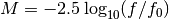• ‘asinh’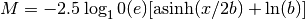astropysics.phot.color_to_Teff(c, colorbands='g-r')

estimate color to effective temperature - currently only g-r is available from McGurk 10 or B-V from Sekiguchi & Fukugita 00

astropysics.phot.color_to_flux_ratio(color, band1, band2)

transforms a color as band1mag-band2mag into a flux ratio flux1/flux2

astropysics.phot.compute_band_zpts(specorscale, bands)

this will use the supplied spectrum to determine the magnitude zero points for the specified bands OR scale the zeropoint

astropysics.phot.cosmo_surface_brightness_correction(Sobs, z, mag=True)

computes

mag determines if mag/as^2 is used or if False, flux

astropysics.phot.distance_from_modulus(dm, ddm=None)

Compute the luminosity distance given the specified distance modulus.

Parameters: dm (scalar or array-like) – distance modulus ddm (scalar or array-like) – distance modulus error luminosity distance in pc if ddm is None, else (d,duppererror,dlowererror)
astropysics.phot.distance_modulus(x, intype='distance', dx=None, autocosmo=True)

Compute the distance modulus given a distance or redshift.

Parameters: x (scalar or array-like) – input redshift or distance intype – Can be: ‘distance’ or ‘d’: treat x as a distance in pc ‘redshift’ or ‘z’: x is treated as a redshift dx – error in the x or None for no error autocosmo (bool) – If True, the cosmological calculation will be automatically performed for z > 0.1. If False, a non-cosmological calculation will be done. If it is ‘warn’, a warning will be issued for any inputs where z>0.1 If dx is None, returns distance modulus (or array of distance moduli). Otherwise, returns (dm,dmuppererror,dmlowererror)
astropysics.phot.flux_ratio_to_color(ratio, band1, band2)

transforms a flux ratio band1flux/band2flux to a color band1mag-band2mag

this converts a flux measurement to a specific intensity assuming the source is a sphere of a specified radius at a specified distance (in the same units)

astropysics.phot.intensities_to_sig(Is, In, exposure=1, area=1)

converts photon count intensities (i.e. photon cm^-2 sr^-1) to signficance values from poisson statistics.

Is is intensity of the signal In is intensity of the background

this converts a specific intensity measurement to a flux assuming the source is a sphere of a specified radius at a specified distance (in the same units)

astropysics.phot.kcorrect(mags, zs, magerr=None, filterlist=['U', 'B', 'V', 'R', 'I'])

Uses the Blanton et al. 2003 k-correction

requires pidly (http://astronomy.sussex.ac.uk/~anthonys/pidly/) and IDL with kcorrect installed

input magnitudes should be of dimension (nfilter,nobj), as should magerr zs should be sequence of length nobj if magerr is None,

returns absmag,kcorrections,chi2s

astropysics.phot.lum_to_mag(L, Mzpt=4.83, Lzpt=1, Lerr=None)

Calculate absolute magnitude from a luminosity.

Parameters: L (scalar, array-like, or mapping.) – The luminosity of the object. If a dictionary, the keys will be taken to specify band names (and Mzpt will be ignored). Mzpt (scalar, array-like, or string) – The magnitude zero point for the band, or a band name. Lzpt (scalar or array-like) – Luminosity units - e.g. 4.64e32 for ergs in V with solar zero points, or 1 for solar. Lerr (scalar or array-like) – Error in luminosity (will be treated with normal approximation) Absolute magnitude if Lerr is None, otherwise (mag,magerr) tuple (error assumes normal approximation).
astropysics.phot.mag_to_lum(M, Mzpt=4.83, Lzpt=1, Merr=None)

Valculate a luminosity from an absolute magnitude.

Parameters: M (scalar, array-like, or mapping.) – Absolute magnitude of the object. If a dictionary, the keys will be taken to specify band names (and Mzpt will be ignored). Mzpt (scalar, array-like, or string) – The magnitude zero point for the band, or a band name. Lzpt (scalar or array-like) – Luminosity units - e.g. 4.64e32 for ergs in V with solar zero points, or 1 for solar. Merr (scalar or array-like) – Error in absolute magnitude Luminosity if Lerr is None, otherwise (lum,lumerr) tuple (error assumes normal approximation).

Note

Mzpt as a string can be ‘U’,’B’,’V’,’R’,’I’,’J’,’H’, or ‘K’ and will use B&M values for solar magnitudes or ‘u’,’g’,’r’,’i’, or ‘z’ from http://www.ucolick.org/~cnaw/sun.html

astropysics.phot.plot_band_group(bandgrp, **kwargs)

Plot a group of bands on the same plot using matplotlib

group can be a sequnce of bands, strings or a dictionary mapping names to bands

kwargs go into matplotlib.pyplot.plot() except for:

• clf

clear figure before plotting

• leg

show legend with band names

• unit

unit to use on plots (defaults to angstroms)

• c

(do not use)

• spec

(do not use)

astropysics.phot.rh_to_surface_brightness(totalm, rh)

Compute the surface brightness given a half-light radius in arcsec. Note that the totalm is the integrated magnitude, not just the half-light

astropysics.phot.set_zeropoint_system(system, bands='all')

this uses a standard system to apply the zeropoints for the specified band names.

system can be: * ‘AB’ - use AB magnitudes * ‘vega’ - use vega=0 magnitudes based on Kurucz ‘93 Alpha Lyrae models * ‘vega##’ - use vega=## magnitudes based on Kurucz ‘93 Alpha Lyrae models

astropysics.phot.teff_to_color(teff, colorbands='g-r')

estimate color to effective temperature - currently only g-r is available from McGurk 10 or B-V from Flower 96

astropysics.phot.transform_dict_ugriz_UBVRI(d, ugrizprimed=False)

Uses UBVRI_to_ugriz() and ugriz_to_UBVRI() to transform a dictionary of magnitudes (or arrays of magnitudes) in one system into the other. The keys in the dictionary should be the name of the bands, while the values are the actual magnitudes. The computed magnitudes will then be added with keys matching the appropriate band name. This uses the Jester 2005 transformation equations.

Parameters: Raises ValueError: d – A dictionary with the names of the magnitudes in one of the systems (not both) are keys. Any missing mags are assumed to be 9999. ugrizprimed (bool) – If True, the ugriz bands are for the SDSS photometric telescope system (u’g’r’i’z’), otherwise, the system of the main photometric survey (ugriz). If either both photometric systems or neither are present in the dictionary.
astropysics.phot.ugriz_to_UBVRI(u, g, r, i, z, ugrizprimed=False)

This function transforms SDSS photometry to the UBVRcIc, using the transformations of Jester et al. (2005).

Parameters: u (scalar or array-like) – u-band magnitude g (scalar or array-like) – g-band magnitude r (scalar or array-like) – r-band magnitude i (scalar or array-like) – i-band magnitude z (scalar or array-like) – z-band magnitude. Note that this is unused in the Jester transform, but is included for completness. ugrizprimed (bool) – If True, the inputs are in the SDSS photometric telescope system (u’g’r’i’z’), otherwise, the system of the main photometric survey (ugriz). (u,g,r,i,z) magnitudes in the requested SDSS system as a tuple of scalars or arrays (depending on the type of the inputs)